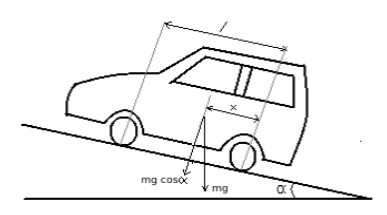# Formula for retardation of car running on level road- Theory of Machines - 1

Q.  If brakes are applied on front wheels of a car and if it moves on a level road, then retardation of the car is calculated using the formula
- Published on 06 Aug 15

a. μ g
b. [μ g (l-x)] / (l + μ h)
c. (μ g x) / (l- μ h)
d. none of the above

ANSWER: (μ g x) / (l- μ h)

#### Discussion

• Amit kumar   -Posted on 20 May 17
Sahi
• Sravanthi    -Posted on 12 Nov 15
1) When brakes are applied on front wheels of a car moving on an inclined plane, the retardation (a) of the vehicle is then given as:

a = (μ g x cos α / l – μ h) + g sinα2) If the vehicle moves on level road, then α = 0

Therefore, retardation of the vehicle is given as:

a = (μ g x) / (l- μ h)

here,
l = distance between rear and front wheels of the vehicle
a = retardation of vehicle
μ = coefficient of friction between wheels and road surface
x = distance of C.G from rear axle

## ➨ Post your comment / Share knowledgeEnter the code shown above:

(Note: If you cannot read the numbers in the above image, reload the page to generate a new one.)